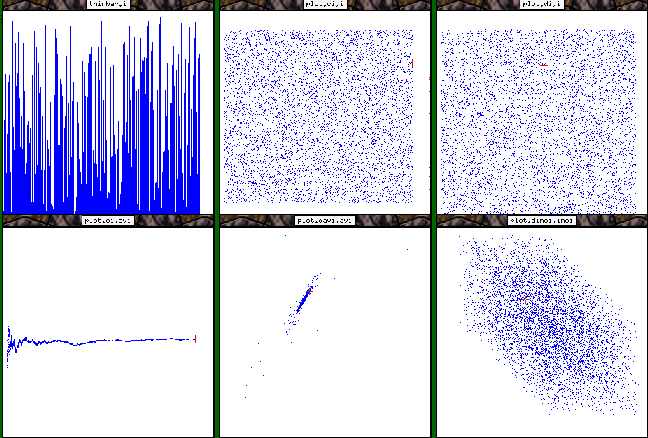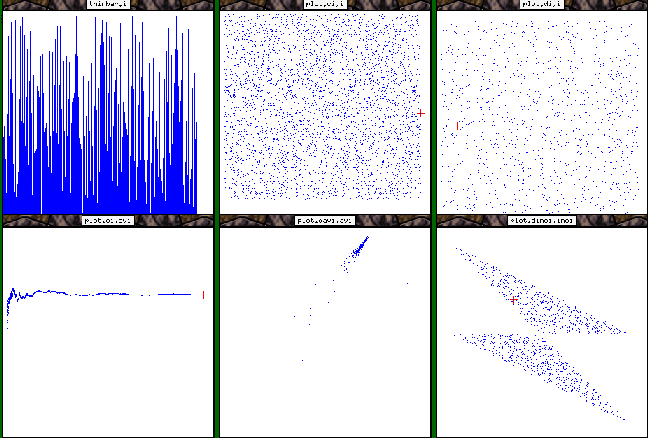# Example of an Algorithm Animation

## The C code

```#include <stdio.h>
#include <math.h>
#include "libAGAT.h"

double drand48(void);

/* Modify this function to change your generator*/
double myRand(void)
{
return drand48();
}

int  main(int argc, char **argv)
{
int             i,j,n;
double          v;

nbn=atoi(argv);
for (i = 1; i <= nbn; i++) {
v = drand48();
agatSendDouble("i", v);
}
}
```
Only the two bold lines have been added for animation. The first one provide the C compiler with prototypes for the agat library functions. The second one send double values 'v' on a stream named 'i' which will be used later in the agat code.

## The Agat code

This code is not exactly a simple one. But you may see that some complex treatments may be achieved in the agat processing step. This simplifies a lot the code of the animated algorithm. Just send everything which may be of some interest in a crude way, then do all refinement in agat.
```--
-- file: aleat.agt
--
include "stat.agt","misc.agt";  -- include some predefined macros

let ci=account(i);      -- count how many values are arrived on stream i
let avi=acaverage(i);   -- average of this values
let inci=increase(i);   -- increase between successives values

let di=delay(i);        -- di is a delayed copy of i
let dci=delay(ci);      -- ...
let davi=delay(avi);
let dinci=delay(inci);

-- and then we visualize all the streams...

-- an histogram view of "i"
thinbar(i);
-- various plots (in different windows)
plot(ci, i);
plot(ci, avi);
plot(di, i);
plot(davi, avi);
plot(dinci, inci);
```

## The result of the execution for different random number algorithmsWith the drand algorithm.With J. von Neumann's algorithm.With Fibonacci's algorithm.

You can easily see the differences between the random generators. The drand algorithm generates good values. There are problems with the others.